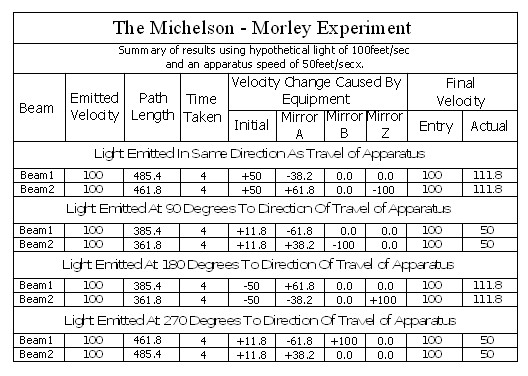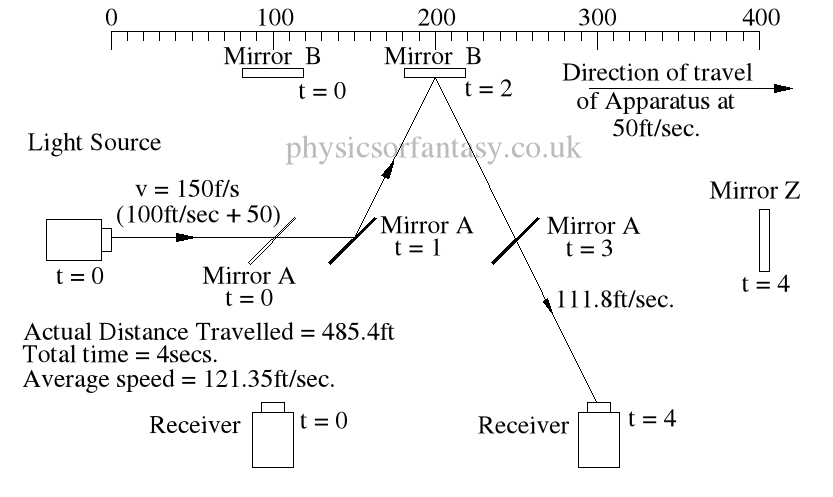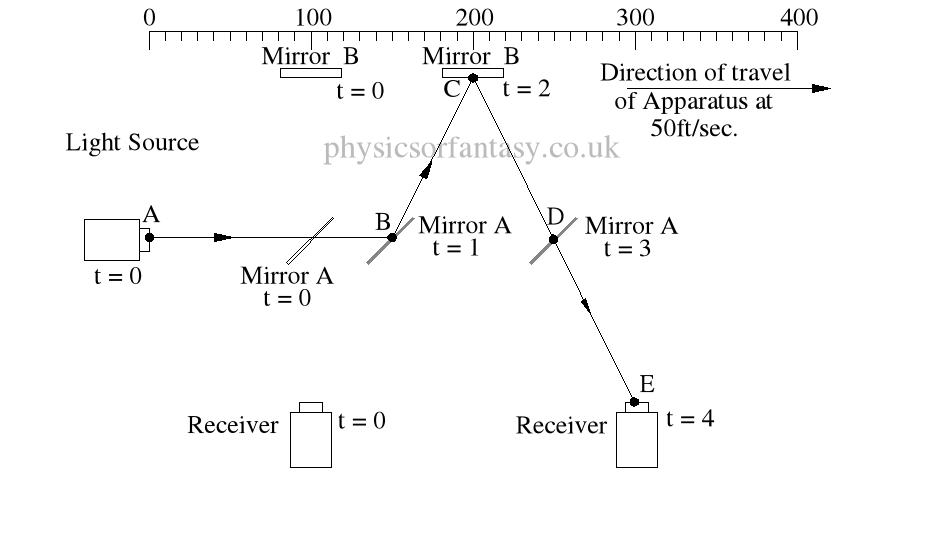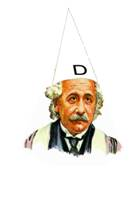An Investigation of Modern Physics by Brian Williams
• ## The Full Mathematics of the Michelson – Morley Experiment. Part 1

Posted on November 28th, 2014

I have never understood why the physics establishment decided that time differences were relevant to the Michelson – Morley experiment. I first looked at this about 48 years ago and my first thoughts were that there could not be any time differences. I am now absolutely certain. The actual results obtained from the experiment are exactly in accordance with the laws and mathematics of classical mechanics.

Basic Set-up of the Experiment.

The idea behind this experiment, dreamed up by Michelson & Morley, was that somehow it would enable them to detect the motion of the Earth in space, (or relative to a fixed point in space), and that it could be detected by differences in the time taken for two beams, travelling different paths, both identical in length. No differences were observed. Note that the physicists assumed that the path lengths were identical. This was the first major error of the Michelson-Morley experiment.

Unfortunately, the experiment (in 1897) was doomed to failure, because the mechanics of it were not understood by Michelson & Morley, (or any later physicists.)

Summary of Actual Mathematics and Mechanics.Note; This is simply an experiment in mechanics, and therefore all the laws of mechanics must be strictly adhered to.

In the above chart ‘Emitted Velocity’ means the velocity that the light travels away from the light source.

Let us consider that the apparatus is travelling in the same direction as the light is emitted.  Let us assume that the speed of light is 100feet/sec, and let us assume that the apparatus is travelling at 50feet/sec.  I do this because using the actual figures leaves us with messy figures such as;

” T2  = (2 x 0.1 x 300,000.0015/300,000) x (1/300,000.0015)” –  From Physics or Fantasy – Section 1. (It would also be difficult to get figures like this into the chart above.)

## Beam 1Within the apparatus the light would travel a distance of 400 feet in 4seconds, travelling from the emitter to Mirror A, then on to Mirror B and then passing through Mirror A again until reaching the Receiver.

However, whilst this is happening, the apparatus will have travelled  200 feet up the laboratory at a velocity of 50feet/second in the same 4 seconds.Ignoring the speed of the light and considering only the speed of the apparatus, the light must pass through the points B, C, D and E, after being emitted from point A, and at the times stated. The distance the light must travel to pass through these points is 485.4 feet. If the apparatus is moving at any speed relative to any point outside the apparatus, then the distance travelled  relative to this external point must be greater or less than the path length within the apparatus, in our example 400 feet.

The light is emitted at time  t = 0, and must arrive at point E because that is where the receiver will be at time t = 4secs.

The velocity of the light relative to its starting position (Or even a fixed point in space) will be 100 ft/sec emission velocity plus 50ft/sec  (the velocity of the apparatus), but only until it strikes Mirror A.

Because Mirror A is moving away from the light, and is at 45° to direction of travel, the reflection of the light is, (according to the laws and mathematics of classical mechanics.) reduced to a velocity of 111.8 ft/sec. In other words the actual velocity of the light is reduced by 38.2 ft/sec.

Anyone who plays tennis, cricket, table tennis etc. will understand why this happens, without needing a degree in physics. A batsman facing a 100mph ball has various options. He can play a forward forcing shot  (which will increase the speed of the ball) over the bowlers head, hopefully, for six runs. He can also move the bat backwards at the time of impact, which will reduce the speed of the ball. In both situations the angle of the bat also affects both the speed and direction of the ball.

#### Mathematics

Va = Velocity of Apparatus.

Ve = Emitted Velocity of the light.

mA = Mirror A, mB = Mirror B, mZ = Mirror Z.

PD =  Physical distance between items of apparatus, example: PD(E>mA) = physical distance between emitter and Mirror A. This is 100ft in all cases in these examples.

TD = Travel distance of light between leaving one item and arriving at the next.

E = Light emitter.

### Beam 1 –  (E>mA)

This is the simplest part of the mathematics.

If the light is emitted at 100ft/sec. and the apparatus is travelling at 50ft/sec then the light must travel at 150ft/sec.

In 1sec. Mirror A will have travelled 50ft from a position 100ft in front of the starting position of the light.

In other words, in 1sec. Mirror A will be 150ft away from the starting position of the light.

If the light travelled a a constant speed of 100ft/sec, it would take 1.5 secs to arrive at the position that Mirror A was after 1 sec. However, it would still be 25 feet behind Mirror A.

### Time taken from Mirror A to Mirror B.

Tan A = Actual distance moved by Mirror B, divided by the physical distance between  Mirror A and Mirror B.

#### TD = Cosine 26.565° = 100/X = 111.8 ft.

It must travel this distance in 1 sec. otherwise it it would miss mirror B. ( Note; Although the mirrors in my diagrams are shown as very wide, in actual practice the mirrors would be narrow, probably about 0.5 inches (12.5mm) wide).

The actual distance travelled by the light from light source to Mirror B is now 150 + 111.8 = 261.8ft. It has taken 2 seconds.

The light now has to travel from mirror B to the receiver R. The actual distance between mirror B and the receiver R is 200ft. However, the light has to travel a distance of 2 x 111.8 to arrive at receiver R.

It takes 2 seconds to travel this distance at a speed of 111.8ft/sec.

### = 485.4ft

The average speed of the light is 485.4/4 = 121.35ft/sec.

It should be obvious that over a period of 4 seconds the light must strike mirror A then Mirror B, then Mirror A again, and finally the receiver R, at the times shown because that is where the items are at the times shown.  The light beam must travel at the speeds shown to allow it strike the items.

Beam 2.

Beam 2 travels from the emitter E to Mirror Z, then back to Mirror B and then onto the Receiver R.

The path length (measured on the equipment) is 400ft.

Note: The light strikes mirror Z at a closing velocity of 100ft/sec, because mirror Z is moving away at 50ft/sec. The light reflection loses another 50ft/sec. because the mirror is still moving away at 50ft/sec.

The speed of the light reflection relative to mirror Z is 100ft/sec. Confusing isn’t it? But it is true. Cricketers, table tennis players, etcetera all use this fact whilst playing their games, and without a honours degree in physics or engineering. Although not an everyday problem in mechanics it does arise quite often in machinery design.

TD(E>mZ)    =       Time taken from Emitter E to Mirror Z.

=       TD(E>mZ)/V2(E>mZ)   =      300/150   =  2 sec.

DT(mZ>mA)  =     Time taken from Mirror Z to Mirror A.

=     TD(mZ>mA)/V(mZ>mA)    =    50/50   =   1 sec.

T(mZ>R)         =       Time taken from Mirror Z to Mirror A.

=       T(mZ>R)/V(mZ>R)   = 111.8/111.8      =   1 sec.

### Notes on lack of time differences in the Michelson – Morley experiment.

If you place the experiment on a train and rotate it through 360° and measure the time taken for the light to travel from the emitter to the the receiver at various angular positions, no time differences will be detected.

——————————————–

If you are in a car travelling eastwards towards the rising Sun at a road speed of 100 mph, then your speed relative to the Sun (It being a ‘fixed point in the universe relative to us), would be approximately 1,100 mph. (The earth rotates at approximately 1000 mph.)

If later the same day on the same road and still travelling at 100 mph, but travelling in a westerly direction towards the setting sun, your speed relative to the sun would be approximately minus 900 mph.  Therefore your speed relative to the sun would have changed by 2000 mph, (i.e. from +1100mph to -900mph.) in the 12 hours between the two journeys.

——————————-

If you replace the car with a beam of  light and carry out similar timings the light will actually be travelling at c + 1000 mph when travelling towards the sunrise, and c-1000mph  when travelling  towards the sunset, ( c =  The claimed constant speed of light.) The velocity of the light (Relative to the Sun) changes by 2000mph between these

Let us change our apparatus to a tube 300,000 kilometres long (approximately). Inside this tube there is a light transmitter(A) at one end and a light receiver(B)  at the other end. We set this tube travelling towards the sun, with end B at the leading end, at a speed of 300,000 kps. When B is at a distance of 300,000 kilometres from the sun we fire the light emmitter at A, towards B (the receiver). One second later end B hits the sun at exactly the same time as the light hits (B). At the time (A) is triggered it is 600,000 kilometres away from the sun.

During this one second the light has travelled 600,000 kilometres and this light has travelled at twice the speed of light!

If we had reversed the tube so that A was at the front end and carried out the same procedure, the light would travel from A to B in two seconds. (end B is still 300,000 kilometres from the sun when end A hits the sun). The light still travels the length of the tube in one second but to an outside observer the light front would be static whilst the end B would be travelling at 300,000 kps towards the light front.

It is this aspect of the Michelson – Morley Experiment that confuses the physicists (plus one or two trigonometry mistakes.)

The man plus the train, the car plus the road, are just different versions of the Michelson – Morley experiment.

Author – Brian Williams

• ## Lightning strikes upwards!

Posted on July 24th, 2010

I was giving informal lectures on this over thirty years ago. Yes, lightning does strike upwards. This was an obvious requirement, as there is a build up of electrons on the surface of the Earth and electricity travels from negative to positive. Visual ‘evidence’ indicates that it travels downwards. High speed photography indicates that lightning travels downwards.. However, the physicists have missed the vital point that the eye or the photography are only seeing the light created by the lightning strike, they are not seeing the actual lightning. An electrical charge is electricity and it is static, lightning is electricity and is moving. The electrical charge on the Earth is static, but under the influence of a positive charge it becomes lightning. The change from a ‘charge’ to lightning requires acceleration, it is not instantaneous. (Basic mechanics)

Now Physics or Fantasy, Section 1 shows conclusively that light does not travel at a constant speed. In fact light travels at various speeds, Blue light travels slower than Red light which travels slower than Yellow light which travels slower than White light. White light travels at a vast range of speeds. The same applies to electricity.

Lightning is accelerating during its passage from the Earth to the clouds. Close to the Earth it is travelling quite slowly, therefore its speed of passage creates only low energy light. On entering the cloud layer it is travelling at its highest speed, and therefore creates a higher energy light. The higher the energy of the light the faster it travels. We therefore see the light created at the cloud height before we see the light created at low level. Therefore the lightning appears to travel downwards. No, this does not prove Einstein’s time dilation, it is just basic mechanics.

The lightning strike passing through the atmosphere my be considered to be like a bullet passing through some very light objects. The bullet scatters the light objects in all directions. The speed of scattering of the light objects is considerably less than the speed of the bullet. Unlike a bullet, lightning is constantly accelerating until it reaches the centre of the positive attraction in the cloud layer. It is therefore almost certain that a lightning discharge travels considerably faster than light.

Recent photographs taken from aircraft travelling above the cloud layers, show lightning apparently striking upwards from the cloud layer. This has created much confusion. However, lightning striking a cloud layer from below may not have all its energy absorbed within the cloud, and part of the energy may continue through it to appear as short lightning ‘strikes’ above the cloud layer. This new evidence is certainly indicative that my early thoughts on this were correct. Obviously I may not be correct, but a logical explanation is better than waffle.

Note;  If lightning is electricity and electricity is electrons, then you should not be able to see lightning if light is photons, unless electrons create photons!!!

Lightning can also occur within the clouds. Although we speak of positive and negative, positive should really be considered as ‘Less Negative’. A negative charge is a a supply of free electrons. These will attempt to redistribute themselves into areas with less electrons, i.e. ‘less negative’ areas.  Clouds will generally have areas that are less negative than others and therefore there will be lightning strikes within the cloud layers.

• ## Einstein’s Wrong Formulae

Posted on June 26th, 2009

Do you know the important differences between these formulae?t1 = [d/(c + v)] + [d/(c – v) ] A1 t1 = [d1/(c + v )] + [d2/(c – v)] A2 t2 = 2d/(c2 – v2)0.5 B1 t2 = (d1 + d2)/(c2 + v2)0.5 B2

Formulae A1 and B1 are the ones used by physicists to ‘prove’ the constant speed of light on the assumption that they are in accordance with classical mechanics. These are the formulae given in physics books. Unfortunately, they are both “special case” formulae which are quite specifically not applicable to the Michelson – Morley experiment on which Einstein’s Relativity is based.

Formulae A2 and B2 are the ones that are correct in accordance with classical mechanics. Although the differences appear minor, Einstein’s Relativity, The Constant Speed of Light, Time Dilation, E = mc2 etc. are all based on the formulae A1 and B1.

More importantly, the above formulae are only 25% of the mathematics needed to solve the problem of the Michelson-Morley experiment. The other 75% is not even considered by Einstein and the rest of the physics establishment

Einstein, not understanding mechanics, did not realise the implications of this error of Michelson and Morley and carried the same error into his own calculations.

If the correct formulae are used i.e. A2 and B2, plus the omitted 75% , all the above hypotheses become extinct, and light is proved to strictly follow the principles of classical mechanics.

Does it matter? Yes, the use of the wrong formulae has cost the people of the UK billions of pounds, and is still costing many millions of pounds every year.

Physics or Fantasy – Section 1 details the errors in full and shows that the mathematics relating to the time of transit for the beams of light in the Michelson – Morley experiment are more complex than realised by the physicists.